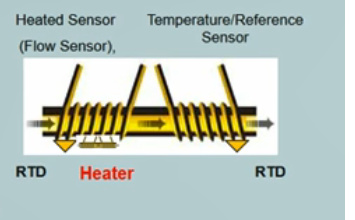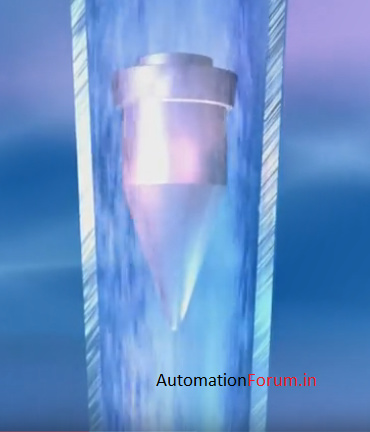# What are different types of gas mass flowmeters?

The gas mass flowmeter measures either the mass velocity at a point in a flowing gas. There are different types of such flow meters. It also measures mass flow, volumetric flow, pressure and temperature in a compact device.

## 1. Laminar Flow Meters

Laminar flow meters use the pressure drop created within a laminar flow element to measure the mass flow rate of a fluid. A laminar flow element takes turbulent flow and separates it into thin channels. By reducing the diameter of the flow channel and affecting the speed, the flow is laminar through the channels. The pressure drop or pressure drop across the channel is measured using a differential pressure sensor. The Poiseuille equation can be used to relate the pressure drop to the volumetric flow rate. The volumetric rate can also be converted to a mass flow using the density correction at a given temperature and pressure.

## 2. Thermal Flow MetersAs the name implies, thermal flow meters use heat to measure the flow velocity of a fluid. Thermal flowmeters traditionally work in one of two ways. The first type measures the current required to maintain a fixed temperature in a heated element. As the fluid flows, the particles come into contact with the element and dissipate or eliminate the heat. As the flow rate increases, more current is needed to maintain the element at a fixed temperature. The current requirement is proportional to the mass flow. The second thermal method involves measuring the temperature at two points in an element or “hot wire”. As the fluid flows over the element, it dissipates the heat. The upstream side of the element will be hotter than the downstream side. The temperature change is related to the mass flow of the fluid.

## 3. Coriolis Flow Meters

The Coriolis flowmeter uses the Coriolis effect to measure the mass flow of a fluid. The fluid travels through single or double curved tubes. Acceleration is applied to the tubes. The Coriolis force acts on the fluid particles perpendicular to the vibration and direction of the flow. While the tube vibrates upward, the fluid flow advances down the tube. As the fluid exits the tube, it forces it upward. This creates torque, twisting the tube. The reverse process occurs when the tube vibrates downward. The amount of rotation in the tube is directly related to the mass flow of the fluid through the tube.

## 4. Ultrasonic flow meters

Ultrasonic flow meters use sound waves to measure the flow of a fluid. Doppler flow meters transmit ultrasonic sound waves to the fluid. These waves are reflected in particles and bubbles in the fluid. The frequency change between the transmitted wave and the received wave can be used to measure the velocity of the fluid flow. Flight time flow meters use the frequency change between transmitted and received sound waves to calculate the velocity of a flow.

## 5. Variable area flow metersThe variable area flow meters, or rotameters, use a tube and float to measure the flow. As the fluid flows through the tube, the float rises. The balance will be reached when the pressure and buoyancy of the float compensate for gravity. The height of the float in the tube is used to reference a flow rate in a calibrated measurement reference.